Ever used an R function that produced a not-very-helpful error message, just to discover after minutes of debugging that you simply passed a wrong argument?

Blaming the laziness of the package author for not doing such standard checks (in a dynamically typed language such as R) is at least partially unfair, as R makes these types of checks cumbersome and annoying. Well, that’s how it was in the past.

Enter checkmate.

Virtually every standard type of user error when passing arguments into function can be caught with a simple, readable line which produces an informative error message in case. A substantial part of the package was written in C to minimize any worries about execution time overhead.

## Intro

As a motivational example, consider you have a function to calculate the faculty of a natural number and the user may choose between using either the stirling approximation or R’s factorial function (which internally uses the gamma function). Thus, you have two arguments, n and method. Argument n must obviously be a positive natural number and method must be either "stirling" or "factorial". Here is a version of all the hoops you need to jump through to ensure that these simple requirements are met:

fact <- function(n, method = "stirling") {
if (length(n) != 1)
stop("Argument 'n' must have length 1")
if (!is.numeric(n))
stop("Argument 'n' must be numeric")
if (is.na(n))
stop("Argument 'n' may not be NA")
if (is.double(n)) {
if (is.nan(n))
stop("Argument 'n' may not be NaN")
if (is.infinite(n))
stop("Argument 'n' must be finite")
if (abs(n - round(n, 0)) > sqrt(.Machine$double.eps)) stop("Argument 'n' must be an integerish value") n <- as.integer(n) } if (n < 0) stop("Argument 'n' must be >= 0") if (length(method) != 1) stop("Argument 'method' must have length 1") if (!is.character(method) || !method %in% c("stirling", "factorial")) stop("Argument 'method' must be either 'stirling' or 'factorial'") if (method == "factorial") factorial(n) else sqrt(2 * pi * n) * (n / exp(1))^n } And for comparison, here is the same function using checkmate: fact <- function(n, method = "stirling") { assertCount(n) assertChoice(method, c("stirling", "factorial")) if (method == "factorial") factorial(n) else sqrt(2 * pi * n) * (n / exp(1))^n } ## Function overview The functions can be split into four functional groups, indicated by their prefix. If prefixed with assert, an error is thrown if the corresponding check fails. Otherwise, the checked object is returned invisibly. There are many different coding styles out there in the wild, but most R programmers stick to either camelBack or underscore_case. Therefore, checkmate offers all functions in both flavors: assert_count is just an alias for assertCount but allows you to retain your favorite style. The family of functions prefixed with test always return the check result as logical value. Again, you can use test_count and testCount interchangeably. Functions starting with check return the error message as a string (or TRUE otherwise) and can be used if you need more control and, e.g., want to grep on the returned error message. expect is the last family of functions and is intended to be used with the testthat package. All performed checks are logged into the testthat reporter. Because testthat uses the underscore_case, the extension functions only come in the underscore style. All functions are categorized into objects to check on the package help page. ## In case you miss flexibility You can use assert to perform multiple checks at once and throw an assertion if all checks fail. Here is an example where we check that x is either of class foo or class bar: f <- function(x) { assert( checkClass(x, "foo"), checkClass(x, "bar") ) } Note that assert(, combine = "or") and assert(, combine = "and") allow to control the logical combination of the specified checks, and that the former is the default. ## Argument Checks for the Lazy The following functions allow a special syntax to define argument checks using a special format specification. E.g., qassert(x, "I+") asserts that x is an integer vector with at least one element and no missing values. This very simple domain specific language covers a large variety of frequent argument checks with only a few keystrokes. You choose what you like best. ## checkmate as testthat extension To extend testthat, you need to IMPORT, DEPEND or SUGGEST on the checkmate package. Here is a minimal example: # file: tests/test-all.R library(testthat) library(checkmate) # for testthat extensions test_check("mypkg") Now you are all set and can use more than 30 new expectations in your tests. test_that("checkmate is a sweet extension for testthat", { x = runif(100) expect_numeric(x, len = 100, any.missing = FALSE, lower = 0, upper = 1) # or, equivalent, using the lazy style: qexpect(x, "N100[0,1]") }) ## Speed considerations In comparison with tediously writing the checks yourself in R (c.f. factorial example at the beginning of the vignette), R is sometimes a tad faster while performing checks on scalars. This seems odd at first, because checkmate is mostly written in C and should be comparably fast. Yet many of the functions in the base package are not regular functions, but primitives. While primitives jump directly into the C code, checkmate has to use the considerably slower .Call interface. As a result, it is possible to write (very simple) checks using only the base functions which, under some circumstances, slightly outperform checkmate. However, if you go one step further and wrap the custom check into a function to convenient re-use it, the performance gain is often lost (see benchmark 1). For larger objects the tide has turned because checkmate avoids many unnecessary intermediate variables. Also note that the quick/lazy implementation in qassert/qtest/qexpect is often a tad faster because only two arguments have to be evaluated (the object and the rule) to determine the set of checks to perform. Below you find some (probably unrepresentative) benchmark. But also note that this one here has been executed from inside knitr which is often the cause for outliers in the measured execution time. Better run the benchmark yourself to get unbiased results. ### Benchmark 1: Assert that x is a flag library(checkmate) library(ggplot2) library(microbenchmark) x = TRUE r = function(x, na.ok = FALSE) { stopifnot(is.logical(x), length(x) == 1, na.ok || !is.na(x)) } cm = function(x) assertFlag(x) cmq = function(x) qassert(x, "B1") mb = microbenchmark(r(x), cm(x), cmq(x)) print(mb) ## Unit: microseconds ## expr min lq mean median uq max neval ## r(x) 3.647 3.757 28.12674 3.8870 4.0375 2402.116 100 ## cm(x) 2.395 2.545 10.58862 2.6295 2.8105 697.423 100 ## cmq(x) 1.603 1.698 12.66232 1.7735 1.8530 1022.480 100 autoplot(mb)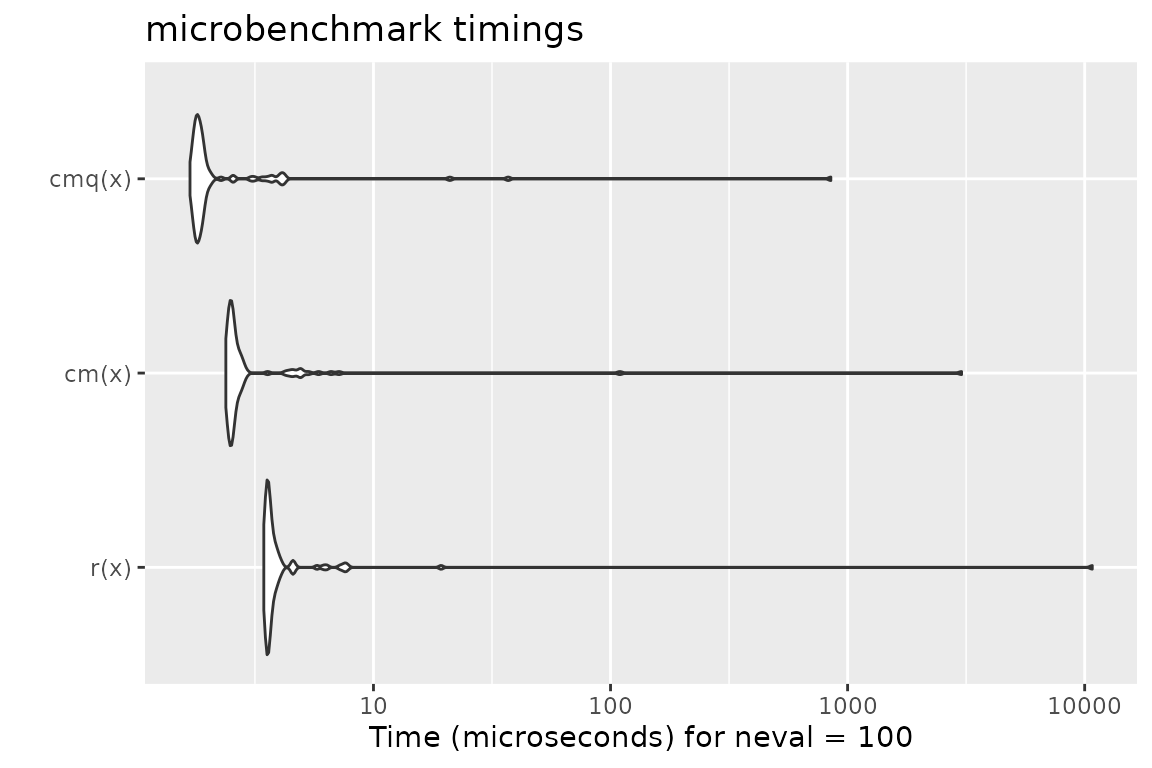### Benchmark 2: Assert that x is a numeric of length 1000 with no missing nor NaN values x = runif(1000) r = function(x) stopifnot(is.numeric(x), length(x) == 1000, all(!is.na(x) & x >= 0 & x <= 1)) cm = function(x) assertNumeric(x, len = 1000, any.missing = FALSE, lower = 0, upper = 1) cmq = function(x) qassert(x, "N1000[0,1]") mb = microbenchmark(r(x), cm(x), cmq(x)) print(mb) ## Unit: microseconds ## expr min lq mean median uq max neval ## r(x) 12.172 12.919 45.22498 13.2345 13.6955 3155.373 100 ## cm(x) 5.510 5.721 16.17575 5.9165 6.1110 912.905 100 ## cmq(x) 6.342 6.502 16.95664 6.5920 6.7230 1029.503 100 autoplot(mb)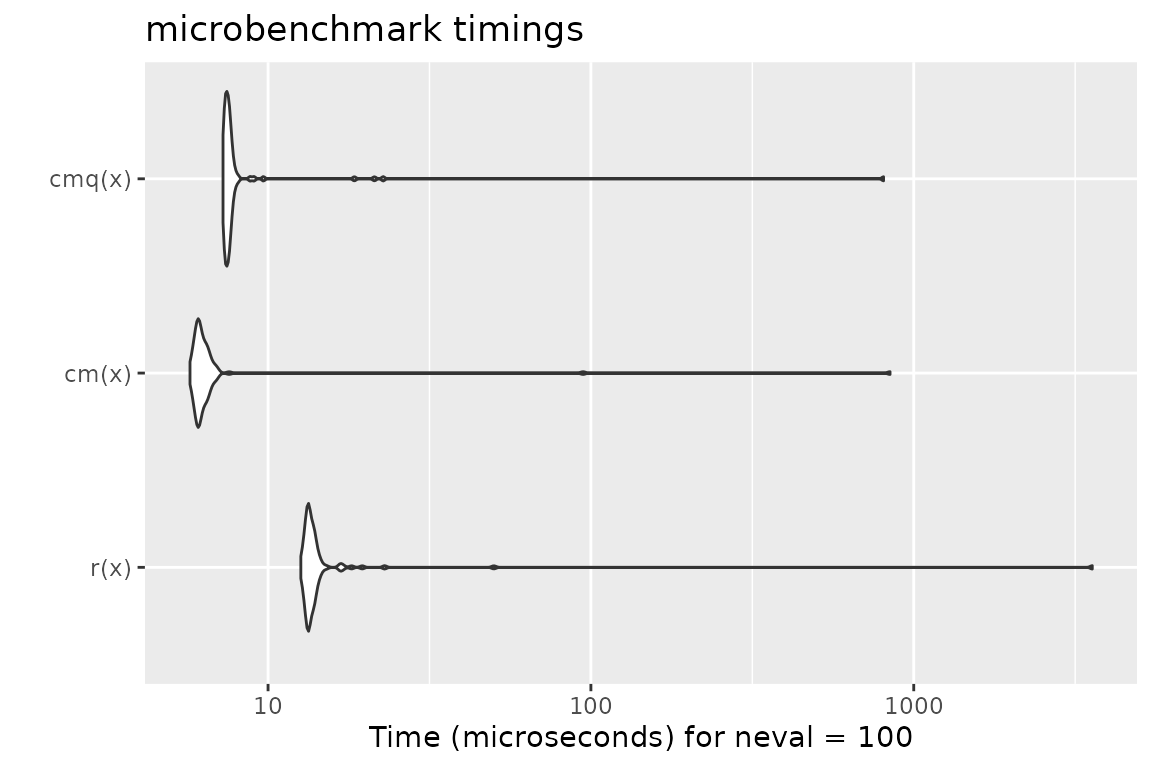### Benchmark 3: Assert that x is a character vector with no missing values nor empty strings x = sample(letters, 10000, replace = TRUE) r = function(x) stopifnot(is.character(x), !any(is.na(x)), all(nchar(x) > 0)) cm = function(x) assertCharacter(x, any.missing = FALSE, min.chars = 1) cmq = function(x) qassert(x, "S+[1,]") mb = microbenchmark(r(x), cm(x), cmq(x)) print(mb) ## Unit: microseconds ## expr min lq mean median uq max neval ## r(x) 281.205 281.8470 319.2710 282.3975 292.0555 2656.231 100 ## cm(x) 289.781 290.5075 302.4056 291.0185 292.1255 1028.011 100 ## cmq(x) 125.205 125.4295 136.5134 125.8605 126.2905 1070.920 100 autoplot(mb)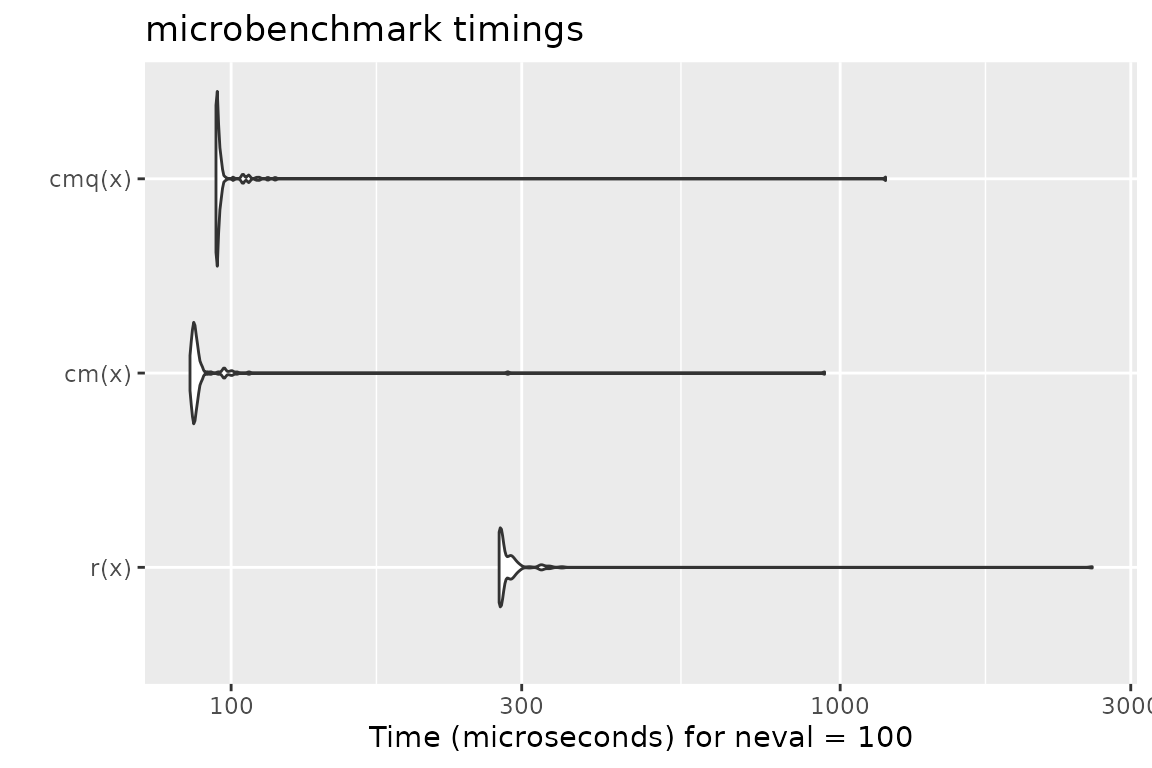### Benchmark 4: Test that x is a data frame with no missing values N = 10000 x = data.frame(a = runif(N), b = sample(letters[1:5], N, replace = TRUE), c = sample(c(FALSE, TRUE), N, replace = TRUE)) r = function(x) is.data.frame(x) && !any(sapply(x, function(x) any(is.na(x)))) cm = function(x) testDataFrame(x, any.missing = FALSE) cmq = function(x) qtest(x, "D") mb = microbenchmark(r(x), cm(x), cmq(x)) print(mb) ## Unit: microseconds ## expr min lq mean median uq max neval ## r(x) 76.272 77.289 106.14803 77.8550 78.5420 2759.253 100 ## cm(x) 35.807 36.583 50.39729 37.7550 38.3415 1058.266 100 ## cmq(x) 28.744 28.929 37.31319 29.3495 29.5455 799.192 100 autoplot(mb)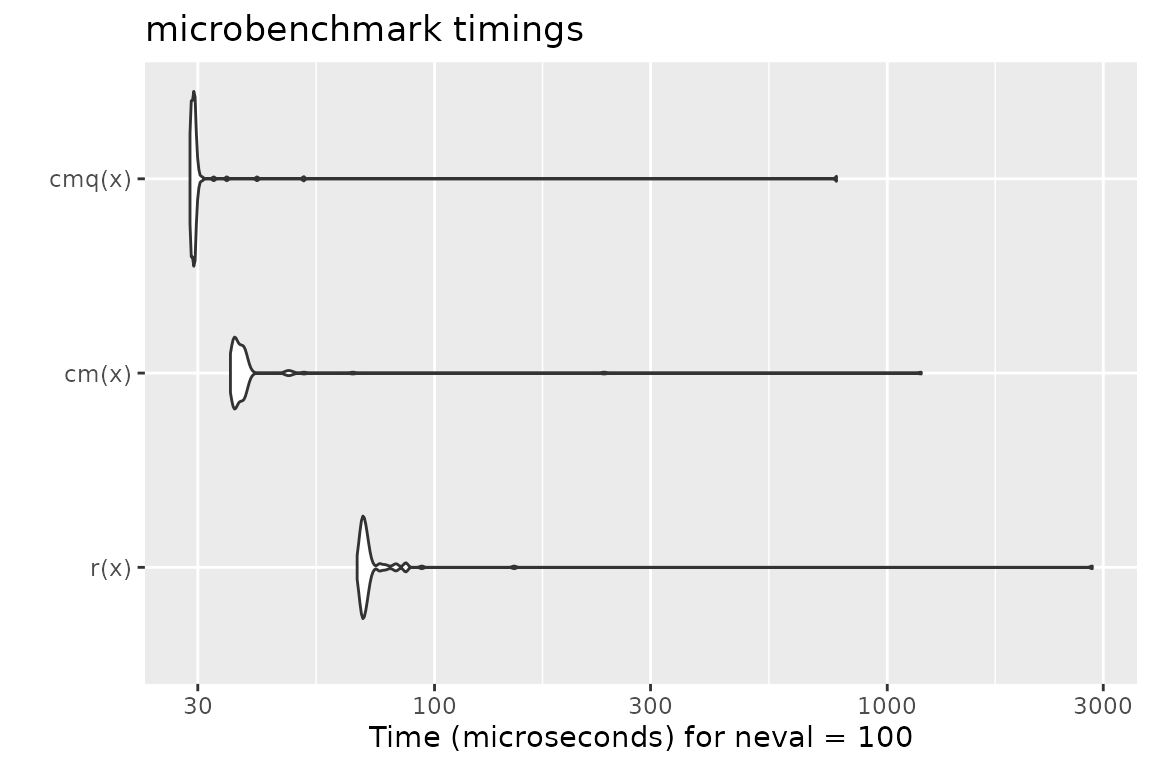# checkmate tries to stop as early as possible x$a = NA
mb = microbenchmark(r(x), cm(x), cmq(x))
print(mb)
## Unit: microseconds
##    expr    min      lq     mean  median      uq     max neval
##    r(x) 62.747 63.9145 68.61822 65.0665 67.7615 134.231   100
##   cm(x)  5.230  5.5410  6.85586  6.6175  7.2590  32.781   100
##  cmq(x)  1.032  1.1620  1.66116  1.3275  1.9630   8.165   100
autoplot(mb)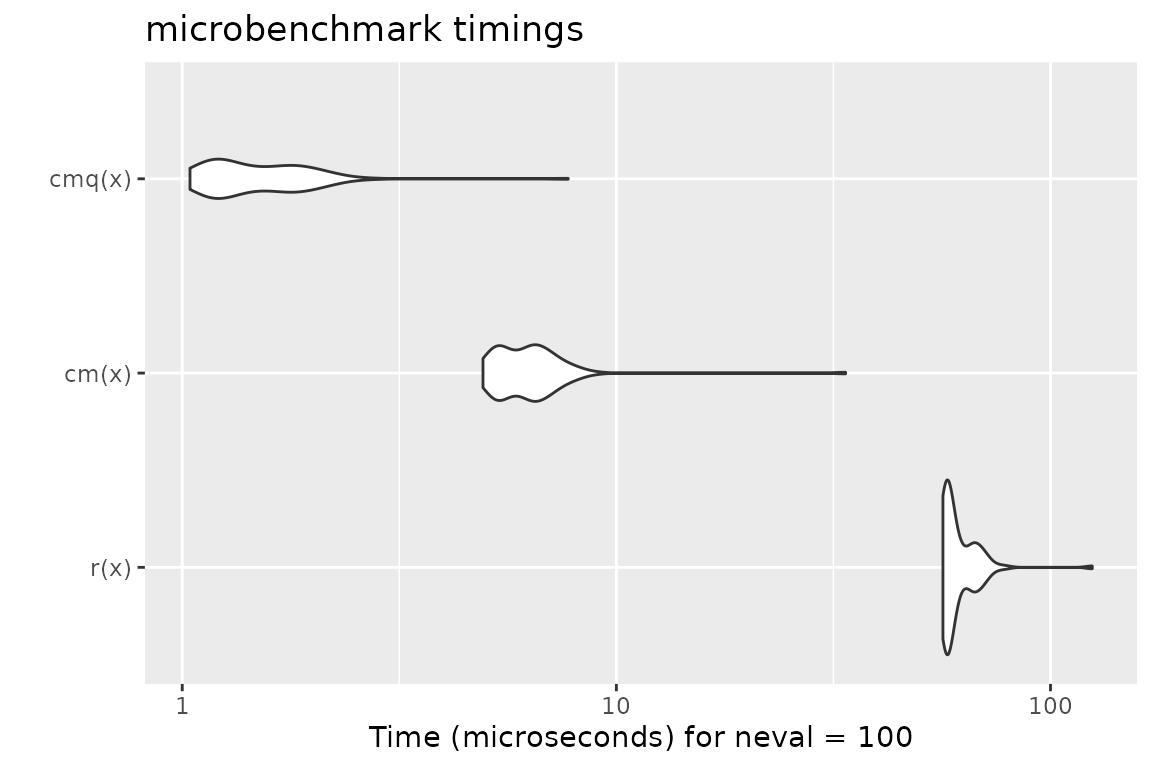### Benchmark 5: Assert that x is an increasing sequence of integers with no missing values

N = 10000
x.altrep = seq_len(N) # this is an ALTREP in R version >= 3.5.0
x.sexp = c(x.altrep)  # this is a regular SEXP OTOH
r = function(x) stopifnot(is.integer(x), !any(is.na(x)), !is.unsorted(x))
cm = function(x) assertInteger(x, any.missing = FALSE, sorted = TRUE)
mb = microbenchmark(r(x.sexp), cm(x.sexp), r(x.altrep), cm(x.altrep))
print(mb)
## Unit: microseconds
##          expr    min      lq     mean  median      uq      max neval
##     r(x.sexp) 29.705 30.0660 53.90406 30.2210 30.4870 2309.253   100
##    cm(x.sexp) 13.015 13.2550 25.24577 13.4455 13.7205 1134.960   100
##   r(x.altrep) 41.929 42.2490 42.76912 42.4445 42.8150   57.898   100
##  cm(x.altrep)  3.687  3.9525  5.52036  4.1180  4.4080  111.008   100
autoplot(mb)## Extending checkmate

To extend checkmate a custom check* function has to be written. For example, to check for a square matrix one can re-use parts of checkmate and extend the check with additional functionality:

checkSquareMatrix = function(x, mode = NULL) {
# check functions must return TRUE on success
# and a custom error message otherwise
res = checkMatrix(x, mode = mode)
if (!isTRUE(res))
return(res)
if (nrow(x) != ncol(x))
return("Must be square")
return(TRUE)
}

# a quick test:
X = matrix(1:9, nrow = 3)
checkSquareMatrix(X)
##  TRUE
checkSquareMatrix(X, mode = "character")
##  "Must store characters"
checkSquareMatrix(X[1:2, ])
##  "Must be square"

The respective counterparts to the check-function can be created using the constructors makeAssertionFunction, makeTestFunction and makeExpectationFunction:

# For assertions:
assert_square_matrix = assertSquareMatrix = makeAssertionFunction(checkSquareMatrix)
print(assertSquareMatrix)
## function (x, mode = NULL, .var.name = checkmate::vname(x), add = NULL)
## {
##     if (missing(x))
##         stop(sprintf("argument \"%s\" is missing, with no default",
##             .var.name))
##     res = checkSquareMatrix(x, mode)
## }
# For tests:
test_square_matrix = testSquareMatrix = makeTestFunction(checkSquareMatrix)
print(testSquareMatrix)
## function (x, mode = NULL)
## {
##     isTRUE(checkSquareMatrix(x, mode))
## }
# For expectations:
expect_square_matrix = makeExpectationFunction(checkSquareMatrix)
print(expect_square_matrix)
## function (x, mode = NULL, info = NULL, label = vname(x))
## {
##     if (missing(x))
##         stop(sprintf("Argument '%s' is missing", label))
##     res = checkSquareMatrix(x, mode)
##     makeExpectation(x, res, info, label)
## }

Note that all the additional arguments .var.name, add, info and label are automatically joined with the function arguments of your custom check function. Also note that if you define these functions inside an R package, the constructors are called at build-time (thus, there is no negative impact on the runtime).

## Calling checkmate from C/C++

The package registers two functions which can be used in other packages’ C/C++ code for argument checks.

SEXP qassert(SEXP x, const char *rule, const char *name);
Rboolean qtest(SEXP x, const char *rule);

These are the counterparts to qassert and qtest. Due to their simplistic interface, they perfectly suit the requirements of most type checks in C/C++.

For detailed background information on the register mechanism, see the Exporting C Code section in Hadley’s Book “R Packages” or WRE. Here is a step-by-step guide to get you started:

1. Add checkmate to your “Imports” and “LinkingTo” sections in your DESCRIPTION file.
2. Create a stub C source file "checkmate_stub.c", see below.
3. Include the provided header file <checkmate.h> in each compilation unit where you want to use checkmate.

File contents for (2):

#include <checkmate.h>
#include <checkmate_stub.c>

## Session Info

For the sake of completeness, here the sessionInfo() for the benchmark (but remember the note before on knitr possibly biasing the results).

sessionInfo()
## R version 4.3.2 (2023-10-31)
## Platform: x86_64-pc-linux-gnu (64-bit)
## Running under: Ubuntu 22.04.3 LTS
##
## Matrix products: default
## LAPACK: /usr/lib/x86_64-linux-gnu/openblas-pthread/libopenblasp-r0.3.20.so;  LAPACK version 3.10.0
##
## locale:
##   LC_CTYPE=C.UTF-8       LC_NUMERIC=C           LC_TIME=C.UTF-8
##   LC_COLLATE=C.UTF-8     LC_MONETARY=C.UTF-8    LC_MESSAGES=C.UTF-8
##  LC_TELEPHONE=C         LC_MEASUREMENT=C.UTF-8 LC_IDENTIFICATION=C
##
## time zone: UTC
## tzcode source: system (glibc)
##
## attached base packages:
##  stats     graphics  grDevices utils     datasets  methods   base
##
## other attached packages:
##  microbenchmark_1.4.10 ggplot2_3.4.4         checkmate_2.3.0
##
## loaded via a namespace (and not attached):
##   gtable_0.3.4      jsonlite_1.8.7    highr_0.10        compiler_4.3.2
##   stringr_1.5.1     jquerylib_0.1.4   systemfonts_1.0.5 scales_1.2.1
##   textshaping_0.3.7 yaml_2.3.7        fastmap_1.1.1     R6_2.5.1
##  knitr_1.45        backports_1.4.1   tibble_3.2.1      desc_1.4.2
##  munsell_0.5.0     rprojroot_2.0.4   bslib_0.6.0       pillar_1.9.0
##  rlang_1.1.2       utf8_1.2.4        cachem_1.0.8      stringi_1.8.1
##  xfun_0.41         fs_1.6.3          sass_0.4.7        memoise_2.0.1
##  cli_3.6.1         withr_2.5.2       pkgdown_2.0.7     magrittr_2.0.3
##  digest_0.6.33     grid_4.3.2        lifecycle_1.0.4   vctrs_0.6.4
##  evaluate_0.23     glue_1.6.2        farver_2.1.1      ragg_1.2.6
##  fansi_1.0.5       colorspace_2.1-0  rmarkdown_2.25    purrr_1.0.2
##  pkgconfig_2.0.3   tools_4.3.2       htmltools_0.5.7